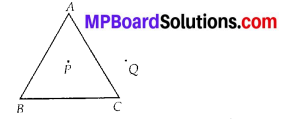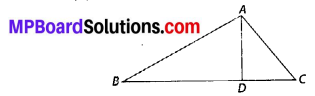# MP Board Class 6th Maths Solutions Chapter 4 Basic Geometrical Ideas Ex 4.4

## MP Board Class 6th Maths Solutions Chapter 4 Basic Geometrical Ideas Ex 4.4

Question 1.
Draw a rough sketch of a triangle ABC. Mark a point P in its interior and a point Q in its exterior. Is the point A in its exterior or in its interior?
Solution:
We have,A is neither in the interior nor in the exterior of the triangle ABC. It is a vertex.Question 2.
(a) Identify three triangles in the figure.
(b) Write the names of seven angles.
(c) Write the names of six line segments.
(d) Which two triangles have ∠B as common?Solution:
(a) The three triangles are ∆ABC, ∆ABD, ∆ADC
(c) 6 line segments are $$\overline{A B}$$, $$\overline{A C}$$, $$\overline{A D}$$, $$\overline{B D}$$, $$\overline{D C}$$, $$\overline{B C}$$